In: Physics

# Light from a He-Ne laser (wavelength 633nm) passes through a single slit of width 25μm. At...

Light from a He-Ne laser (wavelength 633nm) passes through a single slit of width 25μm. At the screen a distance away, the intensity at the center of the central maxima is 8.25 W/m^2.

a. Draw a clear diagram showing the slit and the intensity pattern seen on the screen. Label key quantities and key features.

b. Find the maximum number of totally dark fringes (minima) seen on the screen.

c. At what angle does the dark fringe (minima) that is most distant from the center occur? Note: The small angle approximation will NOT apply.

d. What is the intensity at the peak of the secondary maxima that occurs immediately before the dark fringe in part (b)? Hint: Approximate the angle at which this secondary maxima occurs by assuming it is midway between the angles to the dark fringes on either side of it. Note: The small angle approximation will NOT apply.

## Solutions

##### Expert Solution

(a)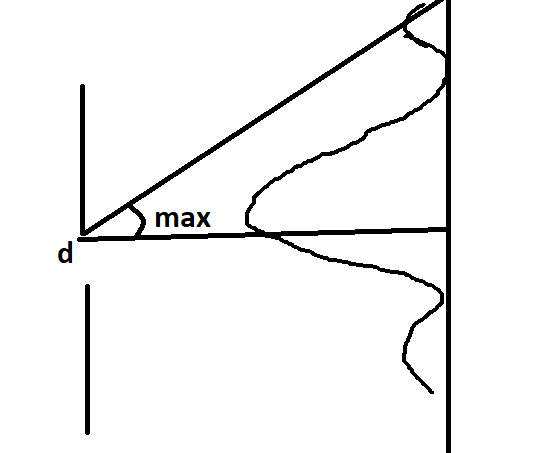_______________________________________

(b)

dsin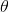= m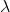where d = 25e-6 m

we want to solve for m, therefore,

m = dsin/To find maximum number of fringes, we need to set= 90

m = 25e-6 * sin 90 / 633e-9

m = 39.49

so,

total number of dark fringes on each side of central maximum is 39 ,

total dark fringes on screen

M = 39 + 39

M = 78

__________________________________

(c)

dsin= mhere, we need to solve for sinwith m = 39 ( most distant fringe)

so,

sin= 39 * 633e-9 / 25e-6

sin= 0.98748= 80.92 degree

so,

distant dark fringe occurs at +/ - 80.9 degree

________________________________________________

(d)

angle for next dark fringe ( m = 38)

sin= 38 * 633e-9 / 25e-6

sin= 0.96216= 74.18 degree

the angle midway is= 80.9 + 74.18 / 2= 77.5 degree

now we find angle for maximum intensity,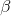/ 2 =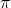dsin// 2 =* 25e-6 * sin 77.5 / 633e-9/ 2 = 121.13 rad

so,

I = Io ( ( sin (/ 2 ) // 2 ) 2

I = 8.25 * (sin 121.13 / 121.13)2

I = 5.445e-4 W/m2

OR

I = 544.5 uW / m2

## Related Solutions

##### In a double-slit experiment, light with a wavelength λ passes through a double-slit and forms an...
In a double-slit experiment, light with a wavelength λ passes through a double-slit and forms an interference pattern on the screen at a distance L from the slits. What statement is true for the resulting interference pattern if the frequency of the light increases? OPTIONS: The distance between maxima stays the same.T he distance between maxima increases. The distance between maxima decreases. Not enough information given.
##### Light of unknown wavelength passes through a double slit, yielding both double slit and diffraction patterns...
Light of unknown wavelength passes through a double slit, yielding both double slit and diffraction patterns on a screen that is 1 m away from the slits. You see that the 9th double-slit maximum coincides with the 2nd single-slit diffraction minimum. You also observe that the first diffraction minimum is located 3 cm from the central axis on the screen. (a) What is the ratio of double-slit separation to single slit width, d/a? (b) If d = 72 µm, what...
##### Light with a wavelength of 616 nm passes through a slit 7.74 μm wide and falls...
Light with a wavelength of 616 nm passes through a slit 7.74 μm wide and falls on a screen 1.90 m away. Q : Find the linear distance on the screen from the central bright fringe to the first bright fringe above it.
##### Blue light of wavelength 470 nm passes through an interference grating with a slit spacing of0.001...
Blue light of wavelength 470 nm passes through an interference grating with a slit spacing of0.001 mm and makes an interference pattern on the wall. How many bright fringes will be seen?
##### Red light of wavelength 675 nm is incident on a slit of width 4.56 × 10−6...
Red light of wavelength 675 nm is incident on a slit of width 4.56 × 10−6 m. An observing screen is placed 1.50 m from the slit. 9a) Find the distance between the third order dark fringe and the central bright fringe (in meters). 9b) If you replaced the single slit with two slits centered on the former position of the single slit, what would the separation between the two slits need to be in order for the second order...
##### Laser light of wavelength 632.8 nm falls normally on a slit that is 0.0230 mm wide....
Laser light of wavelength 632.8 nm falls normally on a slit that is 0.0230 mm wide. The transmitted light is viewed on a distant screen where the intensity at the center of the central bright fringe is 8.80 W/m2. Find the maximum number of totally dark fringes on the screen, assuming the screen is large enough to show them all.At what angle does the dark fringe that is most distant from the center occur?What is the maximum intensity of the...
##### Laser light of wavelength 632.8 nm falls normally on a slit that is 0.0260 mm wide....
Laser light of wavelength 632.8 nm falls normally on a slit that is 0.0260 mm wide. The transmitted light is viewed on a distant screen where the intensity at the center of the central bright fringe is 8.40 W/m^2. Part A Find the maximum number of totally dark fringes on the screen, assuming the screen is large enough to show them all. m_max =    SubmitRequest Answer Part B At what angle does the dark fringe that is most distant...
##### Two thin slits separated by 0.0880 mm are illuminated by light from a He-Ne laser (?...
Two thin slits separated by 0.0880 mm are illuminated by light from a He-Ne laser (? = 633 nm), producing interference fringes on a distant screen. Find the angle between the centers of the central bright fringe and the next bright fringe.
##### Two lasers are sent through the same double-slit device. A blue laser has a wavelength of...
Two lasers are sent through the same double-slit device. A blue laser has a wavelength of 430nm, and a red laser has a wavelength of 645nm. The spacing of the slits is 2mm, and the distance to the screen is 1.0m. A) Sketch what the blue laser will look like on the screen; give a distance scale. B) On your diagram, find a point at which both the red light and blue light will be at a bright fringe. Show...
##### He-Ne lasers are often used in physics demonstrations. They produce light of wavelength 633nm and a...
He-Ne lasers are often used in physics demonstrations. They produce light of wavelength 633nm and a power of 0.650mW spread over a cylindrical beam 1.35mm in diameter (although these quantities can vary). Part A What is the intensity of this laser beam? I=___________W/m^2 Part B What is the maximum value of the electric field? Emax=__________V/m Part C What is the maximum value of the magnetic field? Bmax=__________uT Part D What is the average energy density in the laser beam? Uav=____________J/m^3